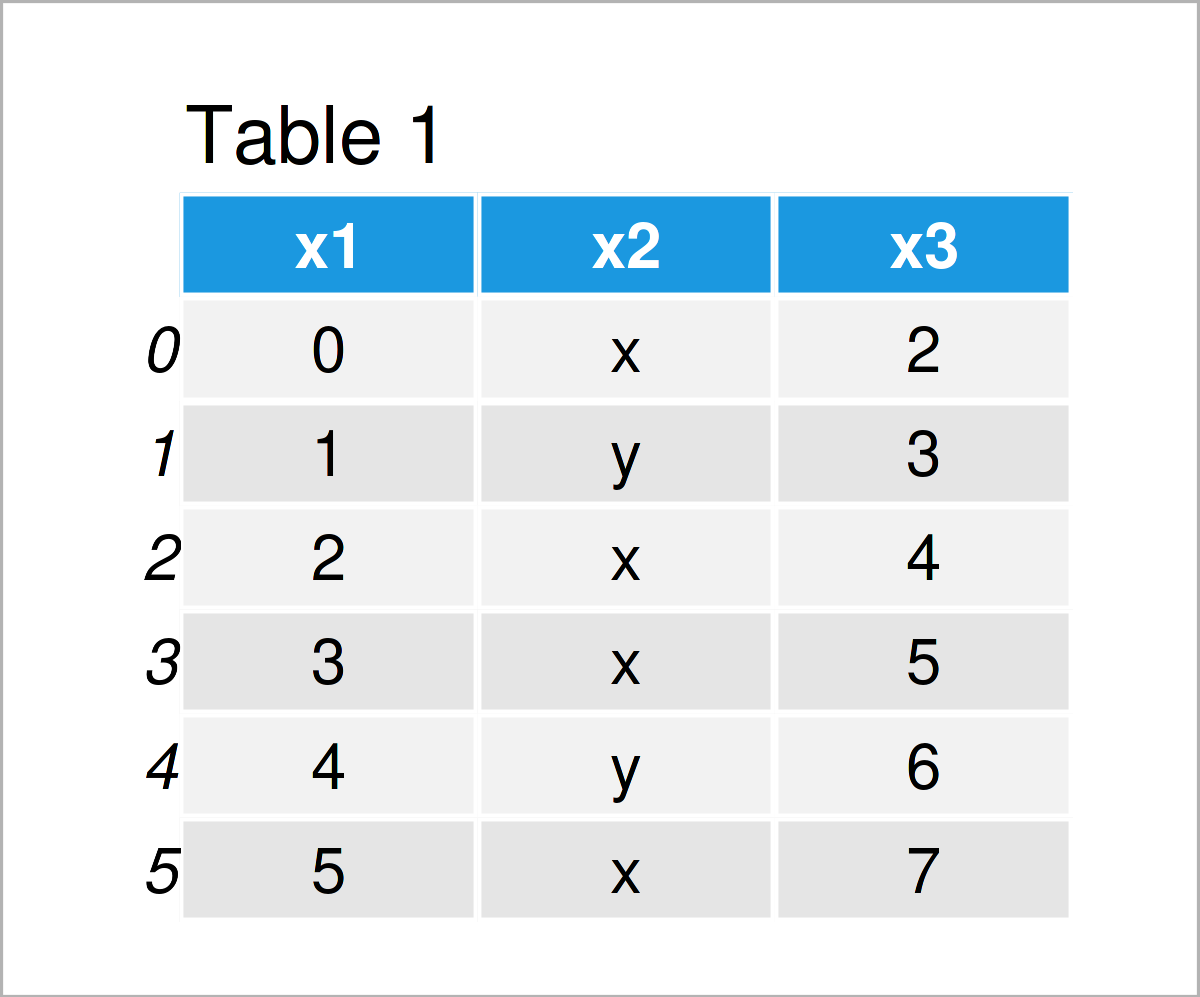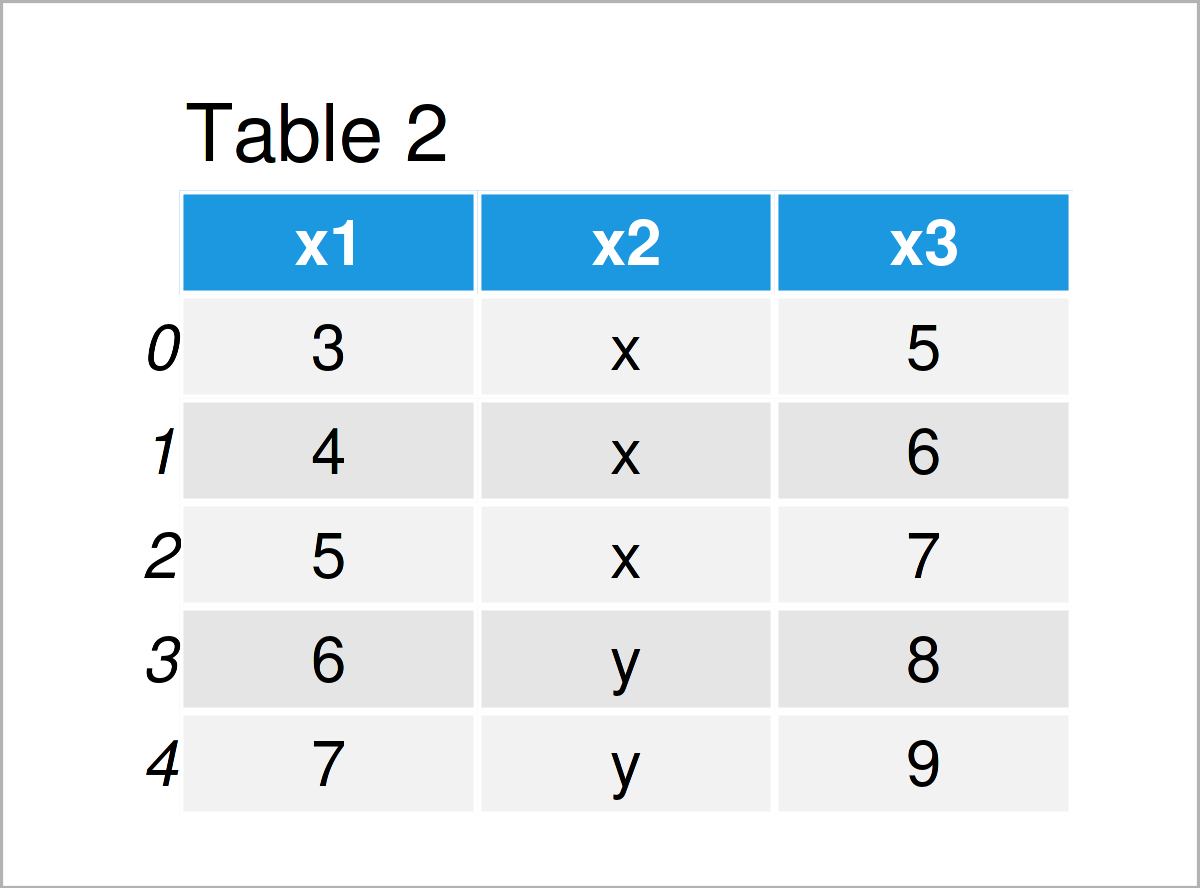# Compare Two CSV Files for Differences in Python (Example)

This post explains how to compare two CSV files and print out differences in Python.

Let’s get started.

## Example Data & Add-On Libraries

We first have to import the pandas library:

`import pandas as pd                                 # Load pandas`

Furthermore, consider the example data below:

```data1 = pd.DataFrame({'x1':range(0, 6),             # Create first pandas DataFrame
'x2':['x', 'y', 'x', 'x', 'y', 'x'],
'x3':range(2, 8)})
print(data1)                                        # Print first pandas DataFrame``````data2 = pd.DataFrame({'x1':range(3, 8),             # Create second pandas DataFrame
'x2':['x', 'x', 'x', 'y', 'y'],
'x3':range(5, 10)})
print(data2)                                        # Print second pandas DataFrame```The output of the previous Python programming syntax is shown in Tables 1 and 2: We have created two pandas DataFrames with the same columns but different values.

```data1.to_csv('data1.csv', index = False)            # Export pandas DataFrames to CSV
data2.to_csv('data2.csv', index = False)```

After the previous Python syntax has been executed, you should find multiple CSV files in your current working directory. These two files will be used as a basis for the following example.

## Example: Compare Two CSV Files & Print Out Differences

The following Python programming syntax shows how to compare and find differences between pandas DataFrames in two CSV files in Python.

More precisely, we are searching for rows that do exist in the second pandas DataFrame, but not in the first DataFrame.

In the first step of this example, we are importing the two pandas DataFrames:

```with open('data1.csv', 'r') as csv1, open('data2.csv', 'r') as csv2:  # Import CSV files

Next, we are using a loop to check for each line in the second CSV file if it exists in the first CSV file. Furthermore, we are creating a new CSV file that contains all rows that exist only in the second CSV file.

Let’s do this:

```with open('data_diff.csv', 'w') as outFile:         # Create CSV file with differences
for row in import2:
if row not in import1:
outFile.write(row)```Table 3 shows the output of the previous Python syntax – A data set showing the differences between the first and the second pandas DataFrame.

Please note that the code of this tutorial is partly based on this Stack Overflow thread. You may have a look there for more details on how to compare different data sets stored in CSV files in Python.

## Video & Further Resources

Have a look at the following video on the Statistics Globe YouTube channel. I illustrate the Python programming syntax of this article in the video.

In addition, you may read some of the related tutorials on this website. A selection of articles is listed here.

This tutorial has demonstrated how to find differences between two CSV files in the Python programming language. If you have additional questions, please let me know in the comments below.

Subscribe to the Statistics Globe Newsletter

•Nav
September 7, 2022 7:52 pm

This solution works well and helps to find the difference between two rows and paste the difference into other file. Nice and clean.

•September 8, 2022 6:42 am

Hey Nav,

This is great to hear, thank you for the kind comment! 🙂

Regards,
Joachim

•Ashutosh Gupta
October 18, 2022 1:03 pm

The code is good but one drawback is there
It also compare the first row means x1 x2 x3

Pls try we skip the first row

•November 14, 2022 12:36 pm

Hey Ashutosh,

Could you please share some code that shows how you would do it?

Regards,
Joachim

•cruzeman
October 21, 2022 8:11 pm

Is there a way to keep the column headings (x1, x2, x3) from being truncated? Since it is in both files, the line “if row not in import1:” removes it from the outFile. How would you go about keeping this in the file?

•November 14, 2022 12:40 pm

Hey Cruzeman,

I’m sorry for the delayed response. I was on a long vacation, so unfortunately I wasn’t able to get back to you earlier. Do you still need help with your question?

Regards,
Joachim

•Rob
November 14, 2022 10:09 pm

Hi Joachim,
The code you stated above removes the same rows which is great but since I have the same headers in both files it also removes that too. How do I get the headers back?
Thanks
Rob

•November 15, 2022 10:32 am

Hey Rob,

My example DataFrames do also contain the same headers and they are not removed. Is there maybe a problem when you import your data?

Regards,
Joachim

•Ricardo
September 2, 2023 2:08 am

Your solution is n*m, where n is the size of the first csv and m is the size of the second one. If you sort both files first and then compare line by line (like a merge operation), the complexity would be nlogn + m, which is O(nlogn) where n>m. This is cheaper than n*m.
Something like:
import1.sort()
import2.sort()
i1, i2 = 0, 0
while i1<len(import1) or i2<len(import2):
if import1[i1] len(import2):
print(” import2[i2] or i1>len(import1)
print(“>”, import1[i1])
i2+=1
else:
i1+=1
i2+=1

•September 4, 2023 8:39 am